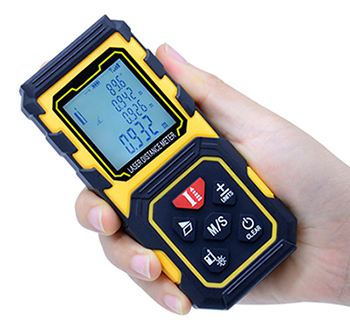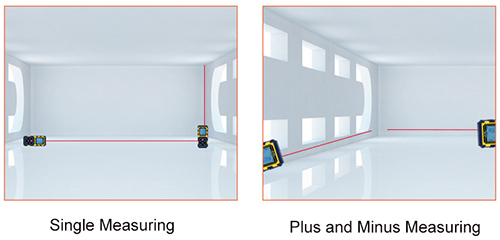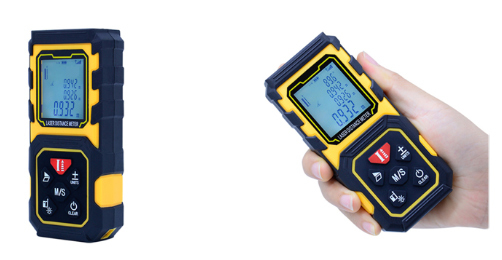Search
All Categories

# Tagged with 'laser distance meter'

FAQ - Laser Distance Meter
1. What is the working principle for laser distance meter? Laser distance meters generally use two methods to measure distance: Pulse method and phase method. The process of pulsed laser ranging is as the follows: The laser light emitted by the laser distance meter is reflected by the object and then received by the laser distance meter, and the round-trip time of the laser will be recorded by the laser distance measurer. So the distance between the laser distance finder and the object can be measured by the speed of light being multiplied by the round-trip time. The accuracy of pulse method measuring distance is generally about ±1 meter. In addition, the measurement blind zone of this type of laser rangefinder is generally about 15 meters.Laser distance measurement and radio detection radars follow the same operating principle: Emit the laser towards the target object, measure the time for the laser to travel back and forth, and calculate the distance by multiplying the recorded time and the velocity of light. The laser is characterized by such merits as high directivity, monochromaticity, and power, which play critical roles in measuring a long distance, judging the target object's direction, raising the reception system's SNR, and guaranteeing the measurement accuracy. With these features, laser distance meters receive growing attention. Laser radars, which have been developed based on laser distance meters, measure not only distances but also the target object's direction, movement velocity, and acceleration.
The measurement effect of a laser distance meter in actual application is roughly determined by four factors, including the size of the target object, the shape of the target object, air quality, and sunlight intensity. If the air quality is high, nearly any laser distance meter can measure the distance of highly-reflective objects, such as a road sign. It is common to hear complaints that a distance meter fails to reach the setting parameter of 1,000m in actual application. Such a problem is frequently mentioned in the laser distance meter industry. In fact, it happens mainly because of users' unfamiliarity with the operating principle of laser distance meters and their inadequate communication with manufacturers.
How does Laser Distance Meter Work?
1. The laser distance meter uses the principle of infrared distance measurement or laser distance measurement. The principle of laser ranging can basically be ascribed to measure the time required for the light to go back and forth to the target, and then the distance D is calculated by the speed of light c=299792458m/s and the atmospheric refraction coefficient n. Because it is difficult to measure time directly, it is usually to measure the phase of continuous wave, which is called phase measuring distance meter. Of course, there are also pulsed distance measures. It should be noted that phase measurement is not to measure the phase of infrared or laser, but to measure the phase of the signal modulated on infrared or laser. The hand-held laser distance meter used for house surveying in the construction industry, applies the same principle.How Accurate is a Laser Distance Meter?
Laser distance meters measure distances by taking lasers as the light source and can be roughly divided into CW laser distance meters and pulsed laser distance meters. Laser distance meters applying gases, including helium-neon, argon ions, and krypton cadmium, work with continuous output and are used for phase-shift laser ranging. Semi-conducting laser distance meters made of DH gallium arsenide are used for infrared distance ranging, while solid laser distance meters made of rubies and neodymium glass are used for pulsed laser ranging.
What is Laser Distance Meter?
The laser distance meter is a measuring tool that accurately determines the distance of the target using a certain parameter of the modulated laser. The pulsed laser distance finder will emit a beam or a sequence of short pulsed laser beams to the target when it is working and receive the laser beam reflected by the target. Then the time from the launch to the reception of the laser beam will be measured by the timer, and the distance between the laser distance measure and the target will be calculated. When the power of the emitted laser beam is sufficient, the range of the laser distance meter can reach about 40 kilometers or more. It can operate day and night, but the distance and accuracy of the ranging will decrease when there are substances with high laser absorption rate in the space.